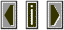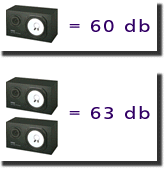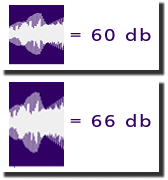DecibelsThe decibel is a measure of the relative intensity or power level of a signal.

 The reference level against which sounds can be measured is the threshold of hearing which is 10**-12 watts/m**2 and is given a value of 0 dB.

 A sound is given a measure in decibels by taking ten times the logarithm of the ratio of its intensity with the intensity of the reference level. 10 log (I/Iref)

 This method of measuring level matches more accurately the psychoacoustical properties of human hearing.

 First of all the dynamic range of human hearing is vast with an effective range spanning the reference level of
10**-12 watts/m**2
up to
10 watts/m**2
which is
10**13
times as intense.
 What this means is that the higher limits of intensity are some 10,000,000,000,000 times as loud as the quietest sound we can hear!

 Secondly the logarithmic nature of this measurement means that if two sounds of the same intensity are added together there will not be a numerical doubling of the intensity measurement but only a 3 dB increase. This is in line with the perception of two such sounds where there is not a perceived doubling of the loudness.doubling of the intensity represents a 3 dB increase. A doubling of the amplitude of a signal will result in a 6 dB increase.